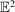International Tables for Crystallography (2016). Vol. A. ch. 3.1, pp. 698-718https://doi.org/10.1107/97809553602060000929

## Contents

• 3.1. Crystal lattices  (pp. 698-719)
• 3.1.1. Bases and lattices  (pp. 698-700) | html | pdf |
• 3.1.1.1. Description and transformation of bases  (p. 698) | html | pdf |
• 3.1.1.2. Lattices  (p. 698) | html | pdf |
• 3.1.1.3. Topological properties of lattices  (p. 698) | html | pdf |
• 3.1.1.4. Special bases for lattices  (pp. 698-699) | html | pdf |
• 3.1.1.5. Remarks  (pp. 699-700) | html | pdf |
• 3.1.2. Bravais types of lattices and other classifications  (pp. 700-708) | html | pdf |
• 3.1.2.1. Classifications  (p. 700) | html | pdf |
• 3.1.2.2. Description of Bravais types of lattices  (pp. 700-701) | html | pdf |
• 3.1.2.3. Delaunay reduction and standardization  (pp. 701-707) | html | pdf |
• 3.1.2.4. Example of Delaunay reduction and standardization of the basis  (pp. 707-708) | html | pdf |
• 3.1.3. Reduced bases  (pp. 709-714) | html | pdf |
• 3.1.3.1. Introduction  (p. 709) | html | pdf |
• 3.1.3.2. Definition  (p. 709) | html | pdf |
• 3.1.3.3. Main conditions  (pp. 709-710) | html | pdf |
• 3.1.3.4. Special conditions  (pp. 710-712) | html | pdf |
• 3.1.3.5. Lattice characters  (pp. 712-713) | html | pdf |
• 3.1.3.6. Applications  (pp. 713-714) | html | pdf |
• 3.1.4. Further properties of lattices  (pp. 714-718) | html | pdf |
• 3.1.4.1. Further kinds of reduced cells  (p. 714) | html | pdf |
• 3.1.4.2. Topological characterization of lattice characters  (pp. 714-715) | html | pdf |
• 3.1.4.3. A finer division of lattices  (p. 715) | html | pdf |
• 3.1.4.4. Conventional cells  (pp. 715-717) | html | pdf |
• 3.1.4.5. Conventional characters  (pp. 717-718) | html | pdf |
• 3.1.4.6. Sublattices  (p. 718) | html | pdf |
• References | html | pdf |
• Figures
• Fig. 3.1.2.1. Conventional cells of the three-dimensional Bravais types of lattices  (p. 700) | html | pdf |
• Fig. 3.1.2.2. Delaunay reduction of Gruber's example (cf  (p. 708) | html | pdf |
• Fig. 3.1.3.1. The net of lattice points in the plane of the reduced basis vectors a and b; OBAD is a primitive mesh  (p. 710) | html | pdf |
• Fig. 3.1.3.2. The effect of the special conditions  (p. 710) | html | pdf |
• Fig. 3.1.3.3. The effect of the special conditions  (p. 711) | html | pdf |
• Fig. 3.1.3.4. The effect of the special conditions  (p. 711) | html | pdf |
• Fig. 3.1.3.5. The effect of the special conditions  (p. 711) | html | pdf |
• Fig. 3.1.4.1. A set M inconsisting of three components  (p. 714) | html | pdf |
• Fig. 3.1.4.2. A convex set in(p. 715) | html | pdf |
• Fig. 3.1.4.3. The Bravais-lattice type of the three-dimensional lattice at the upper end of a line is a limiting case of the type at the lower end  (p. 717) | html | pdf |
• Fig. 3.1.4.4. The Bravais-lattice type of the two-dimensional lattice at the upper end of a line is a limiting case of the type at the lower end  (p. 717) | html | pdf |
• Fig. 3.1.4.5. Three possible decompositions of a two-dimensional lattice L into sublattices of index 2  (p. 718) | html | pdf |
• Tables
• Table 3.1.1.1. Lattice point-group symmetries  (p. 699) | html | pdf |
• Table 3.1.2.1. Two-dimensional Bravais types of lattices  (p. 701) | html | pdf |
• Table 3.1.2.2. Three-dimensional Bravais types of lattices  (pp. 702-703) | html | pdf |
• Table 3.1.2.3. Delaunay types of lattices (`Symmetrische Sorten')  (pp. 704-707) | html | pdf |
• Table 3.1.2.4. Delaunay reduction for Gruber's example  (p. 708) | html | pdf |
• Table 3.1.2.5. Discussion of Gruber's example using the cell surface  (p. 708) | html | pdf |
• Table 3.1.3.1. The parameters,andof the 44 lattice characters ()  (p. 712) | html | pdf |
• Table 3.1.3.2. Lattice characters described by relations between conventional cell parameters  (p. 713) | html | pdf |
• Table 3.1.4.1. Conventional cells for the three-dimensional Bravais types of lattices and their limiting cases  (p. 716) | html | pdf |
• Table 3.1.4.2. Conventional cells for the five two-dimensional Bravais types of lattices and their limiting cases  (p. 717) | html | pdf |
• Table 3.1.4.3. Conventional characters  (p. 718) | html | pdf |